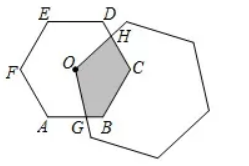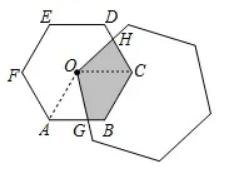$\text{A.}$ $S$ 变化，$l$不变 $\text{B.}$ $S$不变，$l$变化 $\text{C.}$ $S$ 变化, $l$变化 $\text{D.}$ $S$ 与$l$均不变

D

#### 解析：\begin{aligned} & \because \angle H O B=\angle A O C=120^{\circ}, \angle O C H=\angle O A G=60^{\circ}, \\ & \therefore \angle H O C=\angle G O A, \end{aligned}

\begin{aligned} & \left\{\begin{array}{l} \angle H O C=\angle G O A \\ O C=O A \\ \angle O C H=\angle O A G \end{array}\right. \\ & \therefore \triangle H O C \cong \triangle G O A(A S A), \\ & \therefore A G=C H, \end{aligned}

$\therefore S_{\text {阴 }}=S_{\text {四边形 } O A B C}=$ 定值, $l=G B+B C+C H=A G+B G+B C=2 B C=$ 定值,